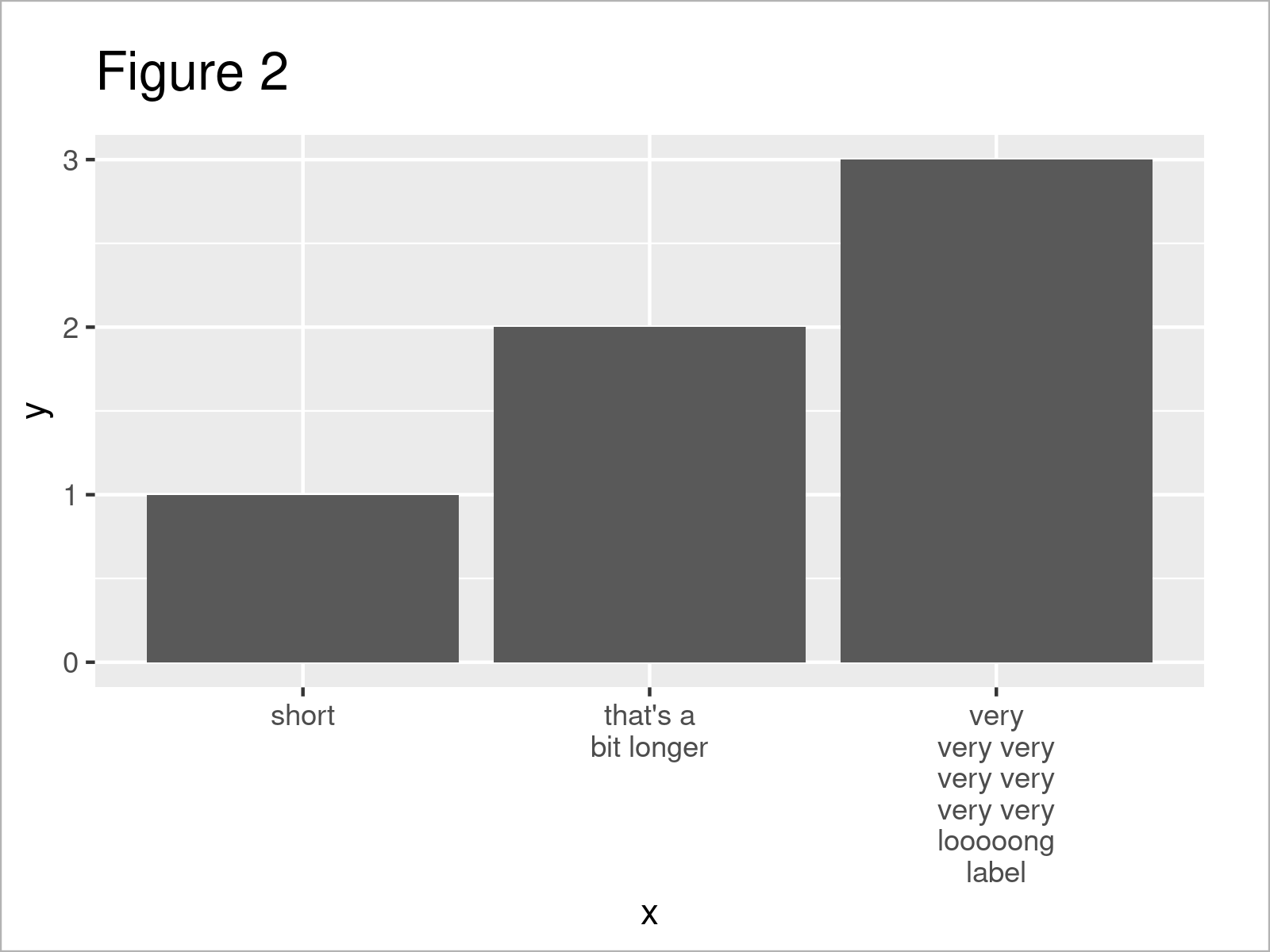# Wrap Long Axis Labels of ggplot2 Plot into Multiple Lines in R (Example)

In this R tutorial you’ll learn how to automatically wrap long axis labels of a ggplot2 graphic.

Here’s the step-by-step process:

## Example Data, Add-On Packages & Basic Graphic

At first, we need to construct some example data:

```data <- data.frame(x = c("short",    # Create example data
"that's a bit longer",
"very very very very very very very looooong label"),
y = 1:3)
data                                 # Print example data
#                                                   x y
# 1                                             short 1
# 2                               that's a bit longer 2
# 3 very very very very very very very looooong label 3```

As you can see based on the previous output of the RStudio console, our example data is a data frame constituted of three rows and the two columns “x” and “y”. The variable x is a character and the variable y has the integer class.

We also need to install and load the ggplot2 package to RStudio, to be able to use the functions that are included in the package:

```install.packages("ggplot2")          # Install ggplot2 package

Now, we can plot our data as follows:

```ggp <- ggplot(data, aes(x, y)) +     # Create ggplot2 barplot
geom_bar(stat = "identity")
ggp                                  # Print ggplot2 barplot```By executing the previous syntax we have created Figure 1, i.e. a ggplot2 barchart with default axis labels. As you can see, the axis labels are very long and are partly overlapping each other.

## Example: Set Maximum Width of ggplot2 Plot Labels Using str_wrap Function of stringr() Package

The following R programming code demonstrates how to wrap the axis labels of a ggplot2 plot so that they have a maximum width.

For this, we first have to install and load the stringr package.

```install.packages("stringr")          # Install stringr package

Now, we can use the str_wrap function of the stringr package to auto wrap the labels of our data to a certain width.

In the following code, we shorten the labels to a maximum width of 10 characters. Whenever this length is exceeded, the label gets broken into a new line.

Have a look at the following R code:

```ggp +                                # Modify labels of ggplot2 barplot
scale_x_discrete(labels = function(x) str_wrap(x, width = 10))```After executing the previously shown R programming syntax the ggplot2 barplot with multi-line labels shown in Figure 2 has been plotted. As you can see, the labels are properly formatted and are not overlapping anymore.

Looks good!

## Video, Further Resources & Summary

Some time ago I have released a video on my YouTube channel, which shows the R programming code of this article. Please find the video below:

Please accept YouTube cookies to play this video. By accepting you will be accessing content from YouTube, a service provided by an external third party.If you accept this notice, your choice will be saved and the page will refresh.

Furthermore, you may read the related tutorials which I have published on https://www.statisticsglobe.com/.

To summarize: You have learned in this article how to automatically wrap too long axis labels of a ggplot2 plot across multiple lines in R programming. If you have further questions and/or comments, let me know in the comments.

Subscribe to the Statistics Globe Newsletter

•Christine Kraamwinkel
January 5, 2022 10:06 am

Hi!

I am working on survey data and the data itself will determine the labels I need on my graph axis. How do I get ggplot to extract those specific labels and then wrap them? I hope my question makes sense! This is my code so far:

ggplot(data=fulldata, aes(y=Health_Months)) +
geom_bar(stat=”count”,fill=”#69b3a2″) +
labs(title=”Household health for the past 12 months”,subtitle=”All households in the pre-test survey”, y=”Frequency”, x = NULL)

•January 5, 2022 10:17 am